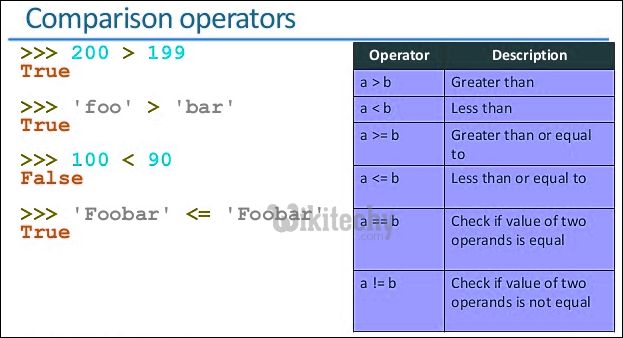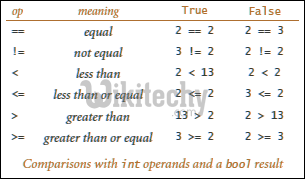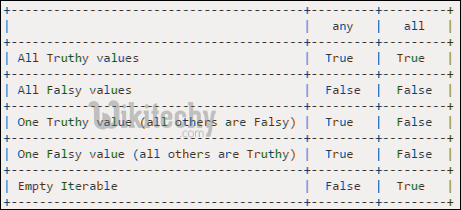# python tutorial - Bitwise Operators | Logical and Bitwise Not Operators on Boolean - learn python - python programmingLearn Python - Python tutorial - python operator - Python examples - Python programs

Most of the languages including C, C++, Java and Python provide the boolean type that can be either set to False or True.Consider below programs that use Logical Not (or !) operator on boolean.

python - Sample - python code :The outputs of above programs are as expected, but the outputs following programs may not be as expected if we have not used Bitwise Not (or ~) operator before.

python - Sample - python code :

python programming - Output :Reason: The bitwise not operator ~ returns the complement of a number i.e., it switches each 1 to 0 and each 0 to 1. Booleans True and False have values 1 and 0 respectively.

˜being the bitwise not operator,

• The expression “˜True” returns bitwise inverse of 1.
• The expression “˜False” returns bitwise inverse of 0.Java doesn’t allow ~ operator to be applied on boolean values. For example, the below program produces compiler error.

python - Sample - python code :

python programming - Output :

Conclusion:
“Logical not or !” is meant for boolean values and “bitwise not or ~” is for integers. Languages like C/C++ and python do auto promotion of boolean to integer type when an integer operator is applied. But Java doesn’t do it.

Wikitechy tutorial site provides you all the learn python , learn python programming , study python programming , python lessons online , python programming for beginner